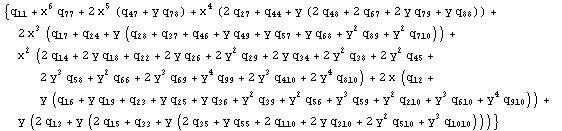# Factor multiple terms in mathematica

• Mathematica
Hello, I have a long equationand using Mathematica 7 I want to factor out terms such as x,y,xy, x^2y,xy^2, etc. so that I'll have it in a form similar to
a + b*x + c*y + d*(xy) + e*(x^2y) + f*(xy^2) + ...
I've tried using the Collect by passing {1,x,y,xy,x^2y,x y^2,...} as the terms to be collected but haven't been successful, I'd appreciate any help!

Thanks!

Hepth
Gold Member
Do you have that equation in Input form so I can play with it?

Also, have you tried just Expand[%]?

Do you have that equation in Input form so I can play with it?
Here you go:
{1, x, y, x^2, y^2, xy, x^3, x^2 y, xy^2,
y^3}.{{Subscript[q, 11], Subscript[q, 12], Subscript[q, 13],
Subscript[q, 14], Subscript[q, 15], Subscript[q, 16],
Subscript[q, 17], Subscript[q, 18], Subscript[q, 19],
Subscript[q, 110]},
{Subscript[q, 12], Subscript[q, 22], Subscript[q, 23],
Subscript[q, 24], Subscript[q, 25], Subscript[q, 26],
Subscript[q, 27], Subscript[q, 28], Subscript[q, 29],
Subscript[q, 210]},
{Subscript[q, 13], Subscript[q, 23], Subscript[q, 33],
Subscript[q, 34], Subscript[q, 35], Subscript[q, 36],
Subscript[q, 37], Subscript[q, 38], Subscript[q, 39],
Subscript[q, 310]},
{Subscript[q, 14], Subscript[q, 24], Subscript[q, 34],
Subscript[q, 44], Subscript[q, 45], Subscript[q, 46],
Subscript[q, 47], Subscript[q, 48], Subscript[q, 49],
Subscript[q, 410]},
{Subscript[q, 15], Subscript[q, 25], Subscript[q, 35],
Subscript[q, 45], Subscript[q, 55], Subscript[q, 56],
Subscript[q, 57], Subscript[q, 58], Subscript[q, 59],
Subscript[q, 510]},
{Subscript[q, 16], Subscript[q, 26], Subscript[q, 36],
Subscript[q, 46], Subscript[q, 56], Subscript[q, 66],
Subscript[q, 67], Subscript[q, 68], Subscript[q, 69],
Subscript[q, 610]},
{Subscript[q, 17], Subscript[q, 27], Subscript[q, 37],
Subscript[q, 47], Subscript[q, 57], Subscript[q, 67],
Subscript[q, 77], Subscript[q, 78], Subscript[q, 79],
Subscript[q, 710]},
{Subscript[q, 18], Subscript[q, 28], Subscript[q, 38],
Subscript[q, 48], Subscript[q, 58], Subscript[q, 68],
Subscript[q, 78], Subscript[q, 88], Subscript[q, 89],
Subscript[q, 810]},
{Subscript[q, 19], Subscript[q, 29], Subscript[q, 39],
Subscript[q, 49], Subscript[q, 59], Subscript[q, 69],
Subscript[q, 79], Subscript[q, 89], Subscript[q, 99],
Subscript[q, 910]},
{Subscript[q, 110], Subscript[q, 210], Subscript[q, 310],
Subscript[q, 410], Subscript[q, 510], Subscript[q, 610],
Subscript[q, 710], Subscript[q, 810], Subscript[q, 910],
Subscript[q,
1010]}} .{{1}, {x}, {y}, {x^2}, {y^2}, {xy}, {x^3}, {x^2 y},
{xy^2}, {y^3}}

Also, have you tried just Expand[%]?
Unfortunately, I've tried it without success.

Thanks for the help!

Hepth
Gold Member
{1, x, y, x^2, y^2, xy, x^3, x^2 y, xy^2,
y^3}
the xy parts need to have spaces "xy"-> "x y" or xy is a new variable.

Also, the second vector, why do you have the double {{},{},{},..}

Code:
f = {1, x, y, x^2, y^2, x y, x^3, x^2 y, x y^2,
y^3}.{{Subscript[q, 11], Subscript[q, 12], Subscript[q, 13],
Subscript[q, 14], Subscript[q, 15], Subscript[q, 16],
Subscript[q, 17], Subscript[q, 18], Subscript[q, 19],
Subscript[q, 110]}, {Subscript[q, 12], Subscript[q, 22],
Subscript[q, 23], Subscript[q, 24], Subscript[q, 25],
Subscript[q, 26], Subscript[q, 27], Subscript[q, 28],
Subscript[q, 29], Subscript[q, 210]}, {Subscript[q, 13],
Subscript[q, 23], Subscript[q, 33], Subscript[q, 34],
Subscript[q, 35], Subscript[q, 36], Subscript[q, 37],
Subscript[q, 38], Subscript[q, 39],
Subscript[q, 310]}, {Subscript[q, 14], Subscript[q, 24],
Subscript[q, 34], Subscript[q, 44], Subscript[q, 45],
Subscript[q, 46], Subscript[q, 47], Subscript[q, 48],
Subscript[q, 49], Subscript[q, 410]}, {Subscript[q, 15],
Subscript[q, 25], Subscript[q, 35], Subscript[q, 45],
Subscript[q, 55], Subscript[q, 56], Subscript[q, 57],
Subscript[q, 58], Subscript[q, 59],
Subscript[q, 510]}, {Subscript[q, 16], Subscript[q, 26],
Subscript[q, 36], Subscript[q, 46], Subscript[q, 56],
Subscript[q, 66], Subscript[q, 67], Subscript[q, 68],
Subscript[q, 69], Subscript[q, 610]}, {Subscript[q, 17],
Subscript[q, 27], Subscript[q, 37], Subscript[q, 47],
Subscript[q, 57], Subscript[q, 67], Subscript[q, 77],
Subscript[q, 78], Subscript[q, 79],
Subscript[q, 710]}, {Subscript[q, 18], Subscript[q, 28],
Subscript[q, 38], Subscript[q, 48], Subscript[q, 58],
Subscript[q, 68], Subscript[q, 78], Subscript[q, 88],
Subscript[q, 89], Subscript[q, 810]}, {Subscript[q, 19],
Subscript[q, 29], Subscript[q, 39], Subscript[q, 49],
Subscript[q, 59], Subscript[q, 69], Subscript[q, 79],
Subscript[q, 89], Subscript[q, 99],
Subscript[q, 910]}, {Subscript[q, 110], Subscript[q, 210],
Subscript[q, 310], Subscript[q, 410], Subscript[q, 510],
Subscript[q, 610], Subscript[q, 710], Subscript[q, 810],
Subscript[q, 910], Subscript[q, 1010]}}.{1, x, y, x^2, y^2, x y,
x^3, x^2 y, x y^2, y^3};
CoefficientList[Expand[f], {x, y}]
F[i_, j_] := CoefficientList[Expand[f], {x, y}][[i+1]][[j+1]];
F[1, 3]

So it makes an array of the coefficients of the powers of x and y. So F[1,3] would be whatever is in front of x y^3.

Thanks!